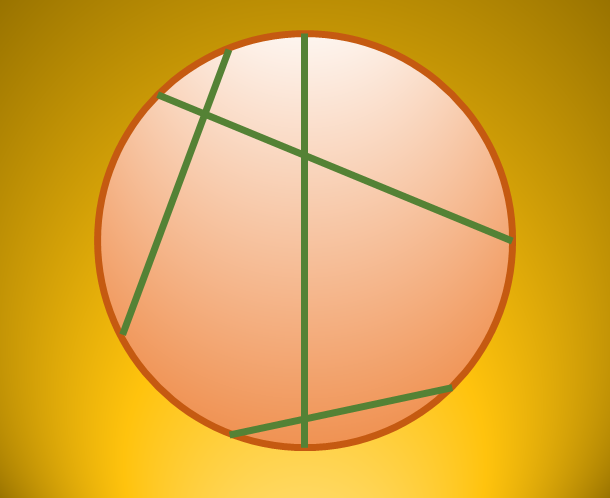Chords chords chordsIn the circumference of a circle of radius $r$, two points are chosen with a uniform distribution. The probability that the length of the chord between these two points is less than $r$ is $\frac{ a}{b}$ for coprime positive integers $a, b$.

Find $a+3b$.

×